## Solution to 1986 Problem 51

The Debye theory and the Einstein theory both describe the vibrational waves in solids. In the Einstein theory, each of the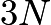$3N$ vibrational modes are treated as independent harmonic oscillators with the same frequency. In the Debye theory, the$3N$ vibrational modes are treated as independent harmonic oscillators with frequencies proportional to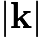$\\left|\\mathbf{k}\\right|$, where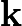$\\mathbf{k}$ is the wavevector. So, answer (B) is correct.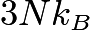$3 N k_B$ is the high temperature limit of the heat capacitiy in both the Debye and the Einstein theory, but in the low temperature limit, the heat capacity is not constant in either theory. So, answer (A) is wrong. In both theories, the crystal is assumed to be composed of atoms arranged in a periodic lattice, so answer (C) is wrong. The Debye theory does not assume anything about the velocities of transverse or longitudinal waves, other than that they are constants. So, answer (D) is wrong. In the Debye theory, there is a cutoff frequency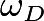$\\omega_D$, but in the Einstein theory, there is one frequency for all of the modes, which is in general different from$\\omega_D$. So, answer (E) is wrong.